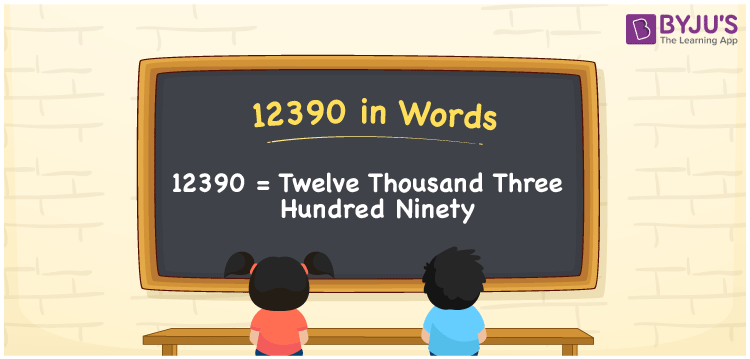# 12390 in Words

12390 in words is Twelve Thousand Three Hundred Ninety. For example, if you saved Rs. 12390 in a month, you can write, “I have saved Rs. Twelve Thousand Three Hundred Ninety in a month”. In general, the number name of any number can be written using the ones, tens, hundreds, and thousands place of a number. Thus, the place value chart is useful for writing the number 12390 in words.

 12390 in words Twelve Thousand Three Hundred Ninety Twelve Thousand Three Hundred Ninety in Numerical Form 12390

## 12390 in English Words## How to Write 12390 in Words?

We can convert 12390 to words using a place value chart. This can be done as follows. The number 12390 has 5 digits, so let’s make a chart that shows the place value up to 5 digits.

 Ten thousands Thousands Hundreds Tens Ones 1 2 3 9 0

Thus, we can write the expanded form as

1 × Ten thousand + 2 × Thousand + 3 × Hundred + 9 × Ten + 0 × One

= 1 × 10000 + 2 × 1000 + 3 × 100 + 9 × 10 + 0 × 1

= 12390

= Twelve Thousand Three Hundred Ninety

12390 is the natural number that is succeeded by 12389 and preceded by 12391.

12390 in words – Twelve Thousand Three Hundred Ninety

Is 12390 an odd number? – No.

Is 12390 an even number? – Yes

Is 12390 a perfect square number? – No

Is 12390 a perfect cube number? – No

Is 12390 a prime number? – No

Is 12390 a composite number? – Yes

## Frequently Asked Questions on 12390 in Words

Q1

### How to write 12390 in words?

12390 in English is written as “Twelve Thousand Three Hundred Ninety”.
Q2

### Is the number 12390 an odd number?

No, the number 12390 is not an odd number.
Q3

### Is 12390 a composite number?

Yes, 12390 is a composite number.
Test your Knowledge on 12390 in Words